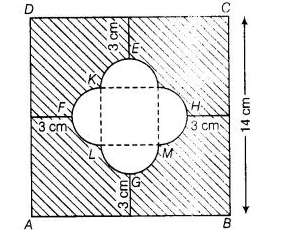# Find the area of the shaded region given in figure

Question:

Find the area of the shaded region given in figureSolution:

Join JK, KL, LM and MJ,

Their are four equally semi-circles and LMJK formed a square.

Their are four equally semi-circles and $L M J K$ formed a square.

$\therefore \quad F H=14-(3+3)=8 \mathrm{~cm}$

So the side of square should be $4 \mathrm{~cm}$ and radius of semi-circle of both ends are $2 \mathrm{~cm}$ each.

$\therefore \quad$ Area of square $J K L M=(4)^{2}=16 \mathrm{~cm}^{2}$

Area of semi-circle $H J M=\frac{\pi r^{2}}{2}$

$=\frac{\pi \times(2)^{2}}{2}=2 \pi \mathrm{cm}^{2}$

$\therefore \quad$ Area of four semi-circle $=4 \times 628=25.12 \mathrm{~cm}^{2}$

Now, $\quad$ area of square $A B C D=(14)^{2}=196 \mathrm{~cm}^{2}$

Area of shaded region =Area of square $A B C D$

- [Area of four semi-circle + Area of square JKLM]

$=196-[8 \pi+16]=196-16-8 \pi$

$=(180-8 \pi) \mathrm{cm}^{2}$

Hence, the required of the shaded region is (180 – 8π)cm².